GET THE APP

Application of Grey Neural Network in Vehicle Vibration Prediction# Advances in Automobile EngineeringOpen Access

Research Article - (2018) Volume 7, Issue 1

# Application of Grey Neural Network in Vehicle Vibration Prediction

Zhendong Zhao* and Rongdong Yin
School of Automobile and Rail Transit, Nanjing Institute of Technology, Nanjing, P.R. China, E-mail: [email protected]
*Corresponding Author: Zhendong Zhao, School of Automobile and Rail Transit, Nanjing Institute of Technology, Nanjing, P.R. China, Tel: +86-25-68533099 Email:

## Abstract

Considering the theoretical characteristics of grey system method and neural network, a grey neural network prediction method was proposed by combining the grey prediction model and the neural network in order to improve the accuracy of vehicle vibration prediction. On the purpose of verifying the proposed model, the vibration data of several measuring points under different traveling speed of a pick-up truck were both tested in an experimental way and predicted in a theoretical way. The results show that it is feasible to apply the proposed grey neural network prediction method to calculate the vehicle vibration data, which improves the prediction accuracy effectively.

Keywords: Grey neural network; Vibrations; GM (1,1) model; Vehicle; Prediction

#### Introduction

Vibration characteristic is one of the most important performances of the vehicle, which affects the comfort of the passengers directly. Vehicle vibration data are generally obtained through experimental or dynamics analysis. In the process of automobile product development, it is necessary to predict vehicle vibration based on incomplete test data. However, the actual vehicle system is nonlinear and complex, in which some information is known and some information is unknown or uncertain. The input-output relationship of a vehicle system is not completely certain or unique, and is like a “quasi-definite” relation. For example, the relation between the power characteristic output and the road input of vehicle presents an uncertain grey domain or grey band, as shown in Figure 1.

Figure 1: A grey relation between the power characteristic output and the road input of vehicle.

Grey prediction model is a nonlinear extrapolation prediction method developed in the 1980s. Because of its practicality, less data required, flexible, convenient modeling and high prediction accuracy, grey prediction model had been widely used in various fields of natural sciences and social sciences.

Artificial neural network has unique self-learning abilities for problems that have necessary data, but it is difficult to be described by an accurate mathematical model. Artificial neural network is able to extract the internal mapping and relation between data after learning and training. The neural network has the advantages of parallel computing, distributed information storage, strong fault-tolerant capability and adaptive learning function, which shows its superior position in dealing with complex artificial intelligence and nonlinear problems.

Both neural network and grey system theory can predict the model parameters, but the two algorithms have their own deficiencies. The combination of grey system theory and neural network algorithm can improve the effectiveness and robustness of prediction . Grey neural network prediction has various combination methods Yuan et al. and Sun et al. [2,3] combined residual amendment grey model with BP neural network, which analyzed small sample time series data. Liu et al.  predicted the rolling force of hot-strip by combining grey theory and BP neural network. Zhang et al.  predicted the wear of cylinder liners by establishing an optimized grey neural network prediction model. Yang et al.  analyzed and predicted the faults of electronic equipment through the construction of grey neural network model. He et al.  took predicting life of gear pump as an example, which proved the improved grey neural network model has higher precision than original GM (1,1) model. In addition, there is another method that grey model is used to predict and then neural network model is used to correct its prediction residual .

In this paper, a new method of predicting vehicle vibration is proposed by combining the grey prediction model and the neural network. The example shows that the method has high prediction accuracy.

#### Methodology

Grey GM (1,1) Model

Grey prediction is usually a prediction method based on GM (1,1) model. GM (1,1) model is a first order univariate differential equation dynamic model with differential, difference, exponential compatibility and other properties. GM (1,1) model mainly deals with the univariate prediction problems . Define a data sequence: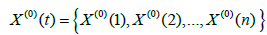Define that X (1) (k) is the 1 cumulative accumulation (1-AGO) of X (0) when t=k, then there is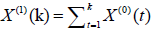(1)

Accumulation can weaken the random interference of original data, and highlight the inherent implication of the system. For generated sequence X (1) (k) , establish the differential equation: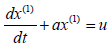(2)

where, a and u are undetermined parameters, and the response functions are obtained by solving the above differential equation: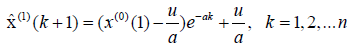(3)

Denote parameter vector as α , then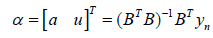(4)

where, B is cumulative generation matrix, yn is a vector,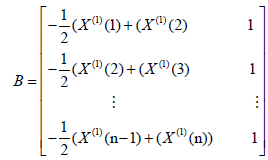(5)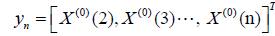(6)

Suppose that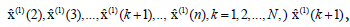is a cumulative value according to equation (3), and reducing value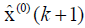is also required to be calculated.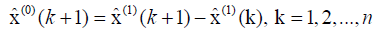(7)

After obtaining the reducing value, the accuracy of the grey model should be tested. The residual error, difference between the reducing value and the actual value, is usually used to verify the accuracy of the model. Then qualified model will be used for prediction.

In this paper, vibration accelerations of all measuring points in a pick-up truck under different speeds were studied applying the proposed method. One measuring points in car body roof and three measuring points in frame close to powertrain mounts were selected for sensor installation in tests. Road vibration test is shown in Figure 2. The installation diagram of vibration acceleration sensor in frame close to transmission is shown in Figure 3. Vibration acceleration test values of all measuring points under different speeds are shown in Table 1 close to engine right mount; Point 4 is in body roof.

Figure 3: The installation diagram of vibration acceleration sensor in frame close to transmission mount.

Vibration
Acceleration/Speed/ Measuring point
30 (km/h) 40 (km/h) 50 (km/h) 60 (km/h) 70 (km/h) 80 (km/h) 90 (km/h)
Point 1 0.658684 0.769572 0.814738 0.945602 1.141909 1.254560 1.705153
Point 2 0.420543 0.449616 0.541690 0.808675 1.186350 1.498909 1.656498
Point 3 0.427067 0.461927 0.533869 0.744361 0.950466 1.285120 1.464960
Point 4 5.391698 6.950552 7.541164 8.291179 10.142348 11.362862 15.876513

Table 1: Vibration acceleration test values of all measuring points under different speeds (m/s2).

GM (1,1) model were established as follows according to Table ① - ④ indexes:

For point 1: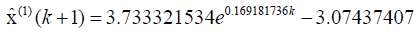(8)

For point 2: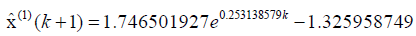(9)

For point 3: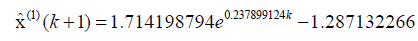(10)

For point 4: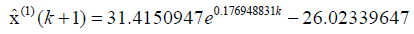(11)

The above models can be applied to the actual prediction after inspection. The vibration accelerations of all vehicle measuring points under 30 km/h-100 km/h speeds were calculated from the model (8) - (11) as shown in Table 2. According to the above Grey prediction method and results, GM (1,1) model only predicts a single data sequence without considering the correlation between each index sequence. The prediction result will be greatly affected when there is disturbance of individual data in the original sequence. So, grey neural network model is introduced to improve the prediction method.

Vibration
Acceleration/Speed/ Measuring point
30 (km/h) 40 (km/h) 50 (km/h) 60 (km/h) 70 (km/h) 80 (km/h) 90 (km/h) 100 (km/h)
Point 1 0.658684 0.688183 0.815040 0.965280 1.143216 1.353951 1.603532 1.899119
Point 2 0.420542 0.503100 0.648024 0.834696 1.075139 1.384846 1.783767 2.297602
Point 3 0.427069 0.460402 0.584057 0.740924 0.939922 1.192367 1.512614 1.918874
Point 4 5.391690 6.081020 7.258123 8.663078 10.339990 12.341502 14.730446 17.581819

Table 2: Vibration acceleration GM (1, 1) predicted values of all measuring points under different speeds (m/s2).

Combined Grey neural network prediction model

The method used in this paper is to establish the GM (1,1) model for four indicator sequences respectively. Then the predictions of the original sequence can be obtained. There may be a deviation between these predicted and actual test values. Moreover, there is mutual coupling between the vibration of these four measuring points. Therefore, neural network model was employed to synthetically analyze the deviation between the predicted value and the actual test value and the mutual coupling relationship between the sequences. The predicted values of GM (1,1) are taken as the input sample of neural network and the actual test values are taken as the output sample. After the neural network is trained by these samples, the weights and thresholds of corresponding nodes can be obtained. Then, the next or more predicted values of GM (1,1) model are taken as inputs of neural network, and the output are the final predicted values.

Multi-layer feedforward neural network based on the error back-propagation algorithm (BP algorithm) was used in the paper. The learning process of BP algorithm is composed of two processes: signal forward propagation and error back propagation. Through the correction of network weight and threshold, the error function is decreased along the gradient direction.

The number of hidden layer neurons is very important for the performance of neural network. If the number of hidden layer neurons is too small, it will not solve the problem. If the number of hidden layer neurons is too much, the tolerance of network will be worse and “overconsistent” will happen easily. The appropriate number of hidden layer neurons is 6 according to the principle of the smallest test error .

Design and analysis of network was carried out based on the BP neural network program compiled in MATLAB. The structure of neural network is shown in Figure 4.

#### Results and Discussion

Simulation and test

The combination method is used to predict the vibration index of vehicle at 100 km/h speed. Then the prediction accuracy is analyzed to demonstrate its feasibility.

Modeling steps are as follows:

1. The GM (1,1) predicted data of four measuring points under 30-90 km/h speeds are taken to construct four data sequences P with length 7;

2. The corresponding actual test data are taken to construct another four data sequences T with length 7;

3. The predicted values (P) are used as the input vector of neural network, and the actual values (T) are used as the output vector of the neural network. The initial weights, thresholds and network structure of the network are initialized;

4. The weights and thresholds of each corresponding node are obtained by training the network;

5. The GM (1,1) predicted values of vehicle at 100 km/h speed are taken as the input of neural network. The corresponding outputs are obtained after simulation, which are the final prediction results of the vibration acceleration of each measuring point at 100 km/h speed. As shown in Table 3, the average of absolute value of the relative error of each sequence was obtained by comparing the predicted results of combined model and GM (1,1) prediction model. The result of combined method is 3.29%, while GM (1,1) model is 6.46%. Absolute value of maximum relative error of combined model is 4.60%, and GM (1,1) model is 9.88%. The superiority of the proposed method in vehicle vibration prediction is shown.

Vibration
Acceleration/Compare/Measuring point
Test Values GM Prediction Model Combined Prediction Method
Predicted
Values
Relative Error
(%)
Predicted
Values
Relative Error
(%)
Point 1 1.805384 1.899119 5.1920 1.7618 2.4141
Point 2 2.174888 2.297602 5.6423 2.0748 4.6020
Point 3 1.746284 1.918874 9.8833 1.6675 4.5115
Point 4 18.532080 17.581819 5.1277 18.8353 1.6362
Average (%)  6.46 3.29

Table 3: Compare results of vibration acceleration values of all measuring points at 100 km/h speed (m/s2).

#### Conclusion

In this paper, the method of predicting vehicle vibration with grey neural network is proposed. The relationship between data within data sequence is considered, and the mutual coupling relationship between data sequences is also considered. The example shows that the combined method has higher prediction accuracy than the single GM (1,1) model. This paper provides a new method for multi-sequence coupled data prediction.

#### Funding

This work was supported by Natural Science Foundation of Jiangsu Province (BK20151462) and Scientific Foundation of Nanjing Institute of Technology (YKJ201432) and “333” Project Foundation of Jiangsu Province (BRA2017449); This work is also sponsored by “Qing Lan “ Project of Jiangsu Province.

#### References

1. Zhang N, Li X, Lu KK (2015) Analysis and research on grey system algorithm combined with neural network (in Chinese). J Marine Electric & Electronic Engineering 35: 28-30.
2. Yuan JL, Zhong L, Li XY (2009) The research and development of grey neural network (in Chinese). J Wuhan University of Technology 31: 91-93.
3. Sun JL, Pang J (2015) Application of Grey neural network based on residual correction in data mining (in Chinese). J Jilin University (Science Edition) 53: 1263-1268.
4. Liu JH, Wang GX, Liu YK (2015) Prediction of rolling force based on grey theory and neural network (in Chinese).J Forging & Stamping Technology 40: 126-129.
5. Zhang XN, Liu AX, Liu B, Zhang HM, Li Y (2011) A study on the wear prediction of engine cylinder liner based on optimized Grey neural network model (in Chinese). J Automotive Engineering 33: 814-817.
6. Yang S, Meng C, Wang C (2013) Research on method of fault prediction based on improved grey neural network (in Chinese). J Application Research of Computers 30: 3625-3628.
7. He QF, Chen GM, Chen XH, Yao CJ (2013) Life prediction of hydraulic pump based on an improved grey neural network (in Chinese). J China Mechnical Engineer 24: 500-506.
8. Dang YG, Liu SF, Wang ZX, Lin Y (2009) Research on Grey prediction and decision model(in Chinese). Beijing Science Press pp.1-15.
9. Wang WC (1998) Neural network and its application in automotive engineering (in Chinese). Beijing Institute of Technology Press, China. pp. 240-245.
Citation: Zhao Z, Yin R (2018) Application of Grey Neural Network in Vehicle Vibration Prediction. Adv Automob Eng 7: 180.

Copyright: © 2018 Zhao Z, et al. This is an open-access article distributed under the terms of the Creative Commons Attribution License, which permits unrestricted use, distribution, and reproduction in any medium, provided the original author and source are credited.
Top